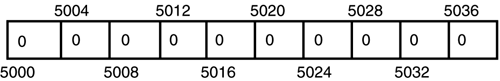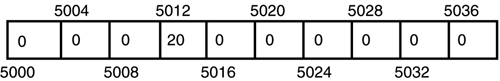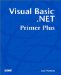# Declaring and Defining Arrays

In Chapter 7, we discussed how you could define and use arrays in your programs. For example, you learned that the statement

` Dim Numbers(10) As Integer `

defines an array of 11 integers. Remember that the number enclosed within the parentheses is the highest index allowed, starting with 0. The number is not the number of elements being defined.

I've also been quite emphatic about the distinction between declaring and defining variables in Visual Basic .NET. The Dim statement defines the Numbers() array because Visual Basic .NET made a call to the Windows memory manager and got enough storage for 11 integer numbers. How much actual storage was given back for the array?

#### Array Scalars

Actually, that's the real purpose of the word Integer in the preceding Dim statement. Integer serves as a scalar that Visual Basic .NET uses to determine how much storage is needed for the array. Because Integer numbers take four bytes each, the scalar for Numbers() is 4. Therefore, Visual Basic .NET needs 44 bytes for the 11 integers in the Numbers() array. In other words, Visual Basic .NET took the number of integers that were requested for the array (that is, 11) and multiplied it by the scalar size of an integer (that is, 4) to determine it needs 44 bytes of memory. Assuming that Windows could find 44 bytes of free memory hanging around, Windows returned the memory address for those 44 bytes to Visual Basic .NET.

#### Array Indexing

After you've defined the Numbers() array, you may use it in a Visual Basic .NET statement. Suppose that you enter the following assignment statement:

` Numbers(3) = 20 `

What does Visual Basic .NET do with this statement? Let's assume that the storage for Numbers() array is 5000. After the definition of the array, it would look something like that shown in Figure 14.1.

##### Figure 14.1. A memory image for the Numbers() array.When Visual Basic .NET processes the assignment statement, the first thing it must to is fetch the memory address for Numbers() . This is memory address 5000 (that is, its lvalue ). Next, Visual Basic .NET takes the array index (or subscript) of the element being used; 3 , in our example. It then takes the scalar value for the Numbers() array, which is 4 for an Integer data type, and multiplies it by the index. The product 12 (that is, 12 = 4 * 3) is then added to the base memory address to get the address where the new rvalue is to be placed. We can show this processing as follows :

` Numbers(3) = 20  lvalue + (index * scalar) = rvalue 5000 + (3 * 4) = 20 5000 + 12 = 20 5012 = 20 `

The assignment statement can be interpreted as the left side of the assignment operator determines where in memory to put what appears on the right side of the assignment operator. Stated more simply, the left side of the assignment operator is "where" to put the "what" from the right side of the operator. When the processing is finished, the Numbers() array looks as shown in Figure 14.2. Notice how the 4 bytes starting at memory address 5012 have changed from Figure 14.1.

##### Figure 14.2. A memory image for the Numbers() array after assignment.It's important for you to understand that indexing into any array is done by calculating an array offset from the base address of the array. The calculation of the array offset is always made using the scalar size of the data item and the index number of the element being used in the expression. Although the math gets a tad more complex, multidimensional arrays use the same array offset method to determine the memory address of a given element.

The good news is that you rarely have to do the indexing math yourself. Visual Basic .NET takes care of all those nasty details for you. However, you should understand how Visual Basic .NET processes array data using array offsets.Visual Basic .NET Primer Plus
ISBN: 0672324857
EAN: 2147483647
Year: 2003
Pages: 238
Authors: Jack Purdum

Similar book on Amazon